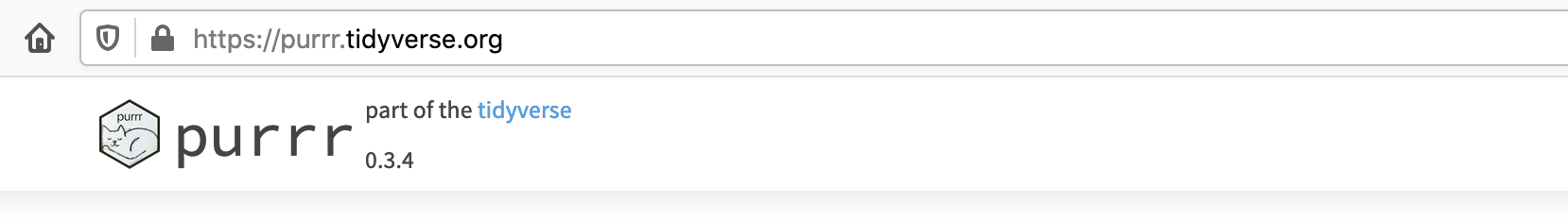Meowr

# Using ellipsis (...) and purrr::pmap()

## Function with arbitrary arguments using ellipsis (…)

Let’s define a function which takes in arbitrary arguments and evaluates an expression on those arguments:

eval_expres <- function(expres, ...){

#this part gets all the ellipsis (...) arguments,
#puts them in a list, and evaluates the rest of the
#function from within the environment defined by that list
with(list(...),

#actual guts of function
eval(parse(text = expres))

)}


Here I chose to explicitly say it needs an argument expres even though it would work with just function(...), because this way, it will throw an error if no expression expres is given.

We can test the function like so:

eval_expres(expres = "2+3")

##  5

eval_expres(expres = "y/x", x = 2, y = pi)

##  1.570796

try(eval_expres())

## Error in parse(text = expres) :
##   argument "expres" is missing, with no default


## Using purrr::pmap() on our function

Let’s make an example data frame to use with the eval_expres() function. Each row is two numbers a and b with an expression expres to evaluate.

library(tidyverse)

df = tibble(a = c(1,2,3),
b = c(5,6,7),
expres = c("a+b","b^2","sqrt(a)"))


We can then use a function from the purrr::pmap() family to evaluate the function multiple times, once for each row of a data frame.

library(purrr)

pmap_dbl(.l = df, .f = eval_expres)

##   6.000000 36.000000  1.732051


Cool!

If you want to learn more about pmap(), check here: https://adv-r.hadley.nz/functionals.html#pmap##### Dr. Gordon McDonald
###### Data Science Group Lead

My research interests include data science for public good, quantum physics and psychology.